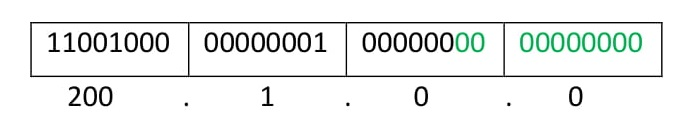GeeksforGeeks App
Open AppBrowser
Continue

Supernetting is the opposite of Subnetting. In subnetting, a single big network is divided into multiple smaller subnetworks. In Supernetting, multiple networks are combined into a bigger network termed as a Supernetwork or Supernet.

Supernetting is mainly used in Route Summarization, where routes to multiple networks with similar network prefixes are combined into a single routing entry, with the routing entry pointing to a Super network, encompassing all the networks. This in turn significantly reduces the size of routing tables and also the size of routing updates exchanged by routing protocols.

More specifically,

• When multiple networks are combined to form a bigger network, it is termed super-netting

• Super netting is used in route aggregation to reduce the size of routing tables and routing table updates

There are some points which should be kept in mind while supernetting:

1. All the Networks should be contiguous.

2. The block size of every network should be equal and must be in form of 2n

3. First Network id should be exactly divisible by whole size of supernet.

Example – Suppose 4 small networks of class C:

```200.1.0.0,
200.1.1.0,
200.1.2.0,
200.1.3.0```

Build a bigger network that has a single Network Id.

Explanation – Before Supernetting routing table will look like as:

First, let’s check whether three conditions are satisfied or not:

1. Contiguous: You can easily see that all networks are contiguous all having size 256 IP Addresses( or 254 Hosts )..
Range of first Network from 200.1.0.0 to 200.1.0.255. If you add 1 in last IP address of first network that is 200.1.0.255 + 0.0.0.1, you will get the next network id which is 200.1.1.0. Similarly, check that all network are contiguous.

2. Equal size of all network: As all networks are of class C, so all of them have a size of 256 which is in turn equal to 28

3. First IP address exactly divisible by total size: When a binary number is divided by 2n then last n bits are the remainder. Hence in order to prove that first IP address is exactly divisible by while size of Supernet Network. You can check that if last n( n here refers to the number of bits required to represent the Total Size of the Supernet ) bits are 0 or not.

In the given example first IP is 200.1.0.0 and whole size of supernet is 4*28 = 210. If last 10 bits of first IP address are zero then IP will be divisible.Last 10 bits of first IP address are zero (highlighted by green color). So 3rd condition is also satisfied.